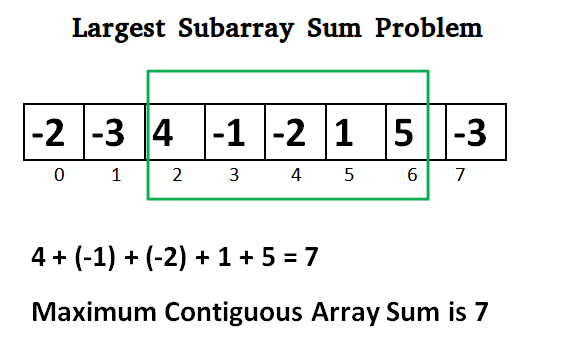Related Articles

# Python Program for Largest Sum Contiguous Subarray

• Last Updated : 12 Dec, 2018

Write an efficient program to find the sum of contiguous subarray within a one-dimensional array of numbers which has the largest sum.## Python

 `# Python program to find maximum contiguous subarray``  ` `# Function to find the maximum contiguous subarray``from` `sys ``import` `maxint``def` `maxSubArraySum(a, size):``      ` `    ``max_so_far ``=` `-``maxint ``-` `1``    ``max_ending_here ``=` `0``      ` `    ``for` `i ``in` `range``(``0``, size):``        ``max_ending_here ``=` `max_ending_here ``+` `a[i]``        ``if` `(max_so_far < max_ending_here):``            ``max_so_far ``=` `max_ending_here`` ` `        ``if` `max_ending_here < ``0``:``            ``max_ending_here ``=` `0`   `    ``return` `max_so_far``  ` `# Driver function to check the above function ``a ``=` `[``-``13``, ``-``3``, ``-``25``, ``-``20``, ``-``3``, ``-``16``, ``-``23``, ``-``12``, ``-``5``, ``-``22``, ``-``15``, ``-``4``, ``-``7``]``print` `"Maximum contiguous sum is"``, maxSubArraySum(a, ``len``(a))``  ` `# This code is contributed by _Devesh Agrawal_`
Output:
```Maximum contiguous sum is -3
```

Above program can be optimized further, if we compare max_so_far with max_ending_here only if max_ending_here is greater than 0.

## Python

 `def` `maxSubArraySum(a, size):``     ` `    ``max_so_far ``=` `0``    ``max_ending_here ``=` `0``     ` `    ``for` `i ``in` `range``(``0``, size):``        ``max_ending_here ``=` `max_ending_here ``+` `a[i]``        ``if` `max_ending_here < ``0``:``            ``max_ending_here ``=` `0``         ` `        ``# Do not compare for all elements. Compare only   ``        ``# when  max_ending_here > 0``        ``elif` `(max_so_far < max_ending_here):``            ``max_so_far ``=` `max_ending_here``             ` `    ``return` `max_so_far`

Please refer complete article on Largest Sum Contiguous Subarray for more details!

My Personal Notes arrow_drop_up